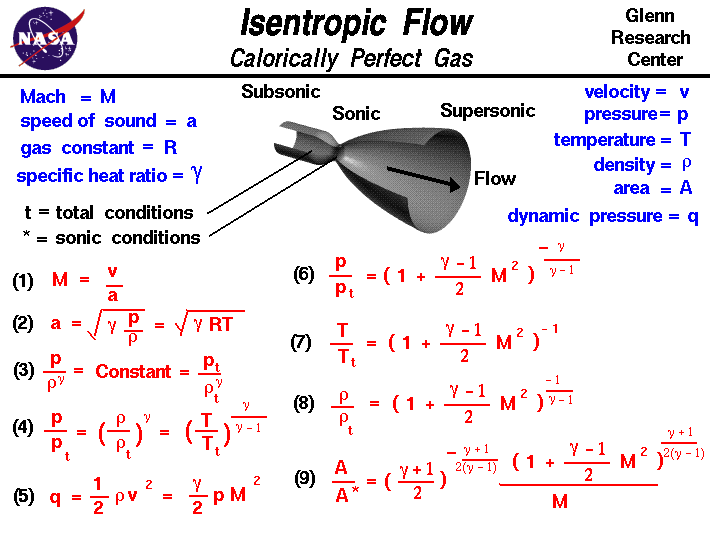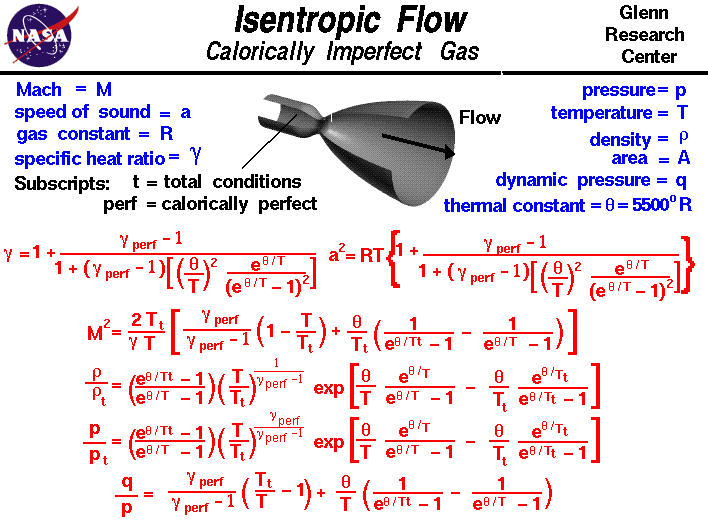+ Text Only Site
+ Non-Flash Version
+ Contact GlennAs a gas is forced through a tube, the gas molecules are deflected by the walls of the tube. If the speed of the gas is much less than the speed of sound of the gas, the density of the gas remains constant and the velocity of the flow increases. However, as the speed of the flow approaches the speed of sound we must consider compressibility effects on the gas. The density of the gas varies from one location to the next. Considering flow through a tube, as shown in the figure, if the flow is very gradually compressed (area decreases) and then gradually expanded (area increases), the flow conditions return to their original values. We say that such a process is reversible. From a consideration of the second law of thermodynamics, a reversible flow maintains a constant value of entropy. Engineers call this type of flow an isentropic flow; a combination of the Greek word "iso" (same) and entropy. Isentropic flows occur when the change in flow variables is small and gradual, such as the ideal flow through the nozzle shown above. The generation of sound waves is an isentropic process. A supersonic flow that is turned while the flow area increases is also isentropic. We call this an isentropic expansion because of the area increase. If a supersonic flow is turned abruptly and the flow area decreases, shock waves are generated and the flow is irreversible. The isentropic relations are no longer valid and the flow is governed by the oblique or normal shock relations. During an isentropic process, the state of the thermodynamic variables of a gas can change. If the specific heat capacity is a constant value, the gas is said to be calorically perfect and if the specific heat capacity changes, the gas is said to be calorically imperfect. At subsonic and low supersonic Mach numbers, air is calorically perfect. But under low hypersonic conditions, air is calorically imperfect. Derived flow variables, like the speed of sound and the isentropic flow relations are slightly different for a calorically imperfect gas than the conditions predicted for a calorically perfect gas because some of the energy of the flow excites the vibrational modes of the diatomic molecules of nitrogen and oxygen in the air. On this page we have collected many of the important equations which describe an isentropic flow. We present two figures and two sets of equations; one for the calorically perfect gas and the other for the calorically imperfect gas. We begin with the calorically perfect gas and the definition of the Mach number since this parameter appears in many of the isentropic flow equations. The Mach number M is the ratio of the speed of the flow v to the speed of sound a. Eq #1: M = v / a The speed of sound, in turn, depends on the density r, the pressure, p, the temperature, T, and the ratio of specific heats gam: Eq #2: a = sqrt(gam * p / r) = sqrt (gam * R * T) where R is the gas constant from the equations of state. If we begin with the entropy equations for a gas, it can be shown that the pressure and density of an isentropic flow are related as follows: Eq #3: p / r^gam = constant We can determine the value of the constant by defining total conditions to be the pressure and density when the flow is brought to rest isentropically. The "t" subscript used in many of these equations stands for "total conditions". (You probably already have some idea of total conditions from experience with Bernoulli's equation). Eq #3: p / r^gam = constant = pt / rt^gam Using the equation of state, we can easily derive the following relations from equation (3): Eq #4: p / pt = (r / rt)^gam = (T / Tt)^[gam/(gam-1)] The dynamic pressure q is defined to be: Eq #5: q = (r * v^2) / 2 = (gam * p * M^2) / 2 Using the conservation of mass, momentum, and energy and the definition of total enthalpy in the flow, we can derive the following relations: Eq #6: p / pt = [1 + M^2 * (gam-1)/2]^-[gam/(gam-1)] Eq #7: T / Tt = [1 + M^2 * (gam-1)/2]^-1 Eq #8: r / rt = [1 + M^2 * (gam-1)/2]^-[1/(gam-1)] Then considering the compressible mass flow equation. we can derive: Eq #9: A / A* = {[1 + M^2 * (gam-1)/2]^[(gam+1)/(gam-1)/2]}*{[(gam+1)/2]^-[(gam+1)/(gam-1)/2]} / M The starred conditions occur when the flow is choked and the Mach number is equal to one. Notice the important role that the Mach number plays in all the equations on the right side of this slide. If the Mach number of the flow is determined, all of the other flow relations can be determined. Similarly, determining any flow relation (pressure ratio for example) will fix the Mach number and set all the other flow conditions. We now turn to the calorically imperfect gas equations.Mathematical models based on a simple harmonic vibrator have been developed for the calorically imperfect gas. The details of the analysis were given by Eggers in NACA Report 959. A synopsis of the report is included in NACA Report 1135. To a first order approximation, the equation for the speed of sound for a calorically imperfect gas is given by: Eq #10: a^2 = R * T * {1 + (gamma - 1) / ( 1 + (gamma-1) * [(theta/T)^2 * e^(theta/T) /(e^(theta/T) -1)^2]) } where gamma is the ratio of specific heats for a perfect gas and theta is a thermal constant equal to 5500 degrees Rankine. The relation for the total temperature is given as: Eq #11: M^2 = (2 (Tt/T) / gam) * [(gamma/(gamma-1) * (1 - T/Tt) + (theta/Tt) * (1/(e^theta/Tt -1) - 1/(e^theta/T -1)] where gam is the ratio of specific heats including a correction for the vibrational modes: Eq #12: gam = 1 + (gamma - 1) / ( 1 + (gamma-1) * [(theta/T)^2 * e^(theta/T) /(e^(theta/T) -1)^2]) The equation relating Mach number and total temperature must be solved iteratively to obtain a value for the total temperature. Having the total temperature ratio, the relations between density and total temperature is: Eq #13: rho/rhot = [(e^(theta/Tt) - 1)/(e^(theta/T) - 1)] * [(T/Tt)^(1/(gamma-1))] * exp[(theta/T) * (e^(theta/T)/(e^(theta/T) -1)) - (theta/Tt) * (e^(theta/Tt)/(e^(theta/Tt) -1)) ] where rho/rhot is the ratio of the static density to the total density. There is a similar relation for the ratio of the static and total pressure p/pt: Eq #14: p/pt = [(e^(theta/Tt) - 1)/(e^(theta/T) - 1)] * [(T/Tt)^(gamma/(gamma-1))] * exp[(theta/T) * (e^(theta/T)/(e^(theta/T) -1)) - (theta/Tt) * (e^(theta/Tt)/(e^(theta/Tt) -1)) ] The equation for the dynamic pressure coefficient q/p is given by: Eq #15: q/p = (gamma/(gamma-1)) * (Tt/T - 1) + (theta/T)*[1/(e^(theta/Tt) -1) - 1/(e^(theta/T) -1) ] Here is a Java program that solves the equations given on this page. This page shows an interactive Java applet to learn how isentropic flows behave by varying the individual flow variables. You select an input variable by using the choice button labeled Input Variable. Next to the selection, you then type in the value of the selected variable. When you hit the red COMPUTE button, the output values change. Some of the variables (like the area ratio) are double valued. This means that for the same area ratio, there is a subsonic and a supersonic solution. The choice button at the right top selects the solution that is presented. The variable "Wcor/A" is the corrected airflow per unit area function which can be derived from the compressible mass flow. This variable is only a function of the Mach number of the flow. The Mach angle and Prandtl-Meyer angle are also functions of the Mach number. These additional variables are used in the design of high speed inlets, nozzles and ducts. If you are an experienced user of this calculator, you can use a sleek version of the program which loads faster on your computer and does not include these instructions. You can also download your own copy of the program to run off-line by clicking on this button: Activities: Guided Tours Navigation ..Beginner's Guide Home Page+ Inspector General Hotline + Equal Employment Opportunity Data Posted Pursuant to the No Fear Act + Budgets, Strategic Plans and Accountability Reports + Freedom of Information Act + The President's Management Agenda + NASA Privacy Statement, Disclaimer, and Accessibility CertificationEditor: Tom Benson NASA Official: Tom Benson Last Updated: Jun 12 2014 + Contact Glenn